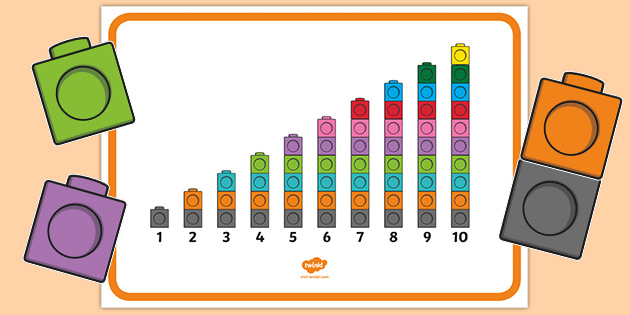# Maths

## Counting in 10s

Welcome back Year one!

This term our learning focus will be addition and subtraction.

We will:

 Understand the vocabulary related to addition (+), subtraction (−) and equals (=) signs.

 Represent and use number bonds and related subtraction facts within 10.

 Solve one-step problems that involve addition and subtraction, using concrete objects.## We have been using Numicon to help us add!

We will be starting the year off by studying place value.

We will be:

 Continue to count from any number to 100.

 Count and read numbers to 100 in numerals.

Understand that the position a digit is placed in a number determines its value.

 Order numbers correctly to 30.

 Identify numbers using objects and use the language of: more than, less than (fewer), most, least.

 Read and write numerals and number words from 1-10.